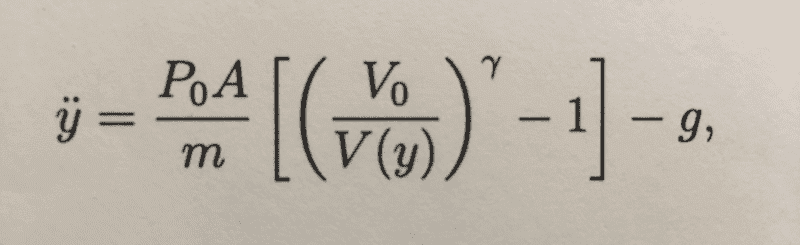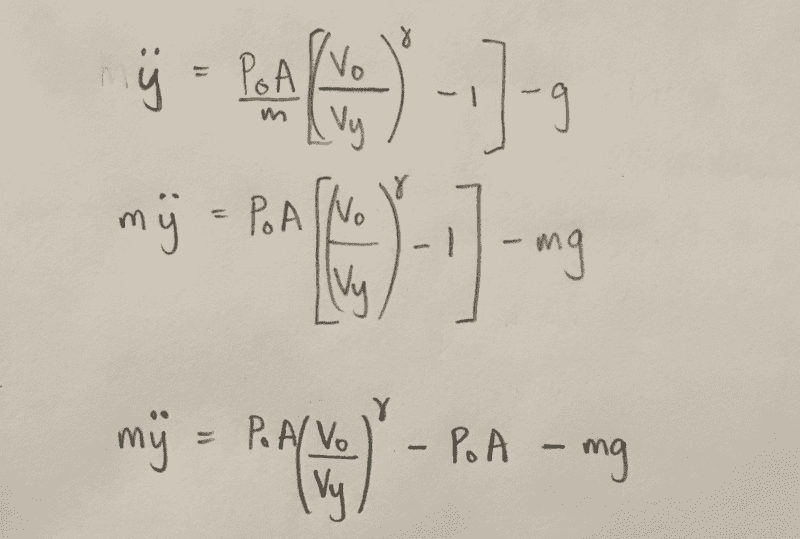# Piston in a tube connected to a vessel filled with air

• I
Hi there, i have been working with a problem the past few days and have a hard time with some of the assumptions being made.
The problem involves a piston in a tube which is connected to a vessel filled with air.
The air behaves as an ideal gas to which the product PV^ζ is a constant.
The piston is released from y=0 at t=0 and we are asked to show that the equation of motion for the piston can be expressed as the attached photo shows:V(y) is gas volume when piston is at y position. There is no friction.

I don't understand what the [([V][/0]/[V[/y])[/ζ] is and how it came to be like this.

this is where i am at with it:if you could provide some insight to what the force on the piston from the vessel involves it would be much appreciated.

Thank you :)

## Answers and Replies

dRic2
Gold Member
Since ##PV^{\gamma} = const## then $$P_0V_0^{\gamma} = PV^{\gamma} \rightarrow P = P_0 \left( \frac {V_0}{V} \right) ^{\gamma}$$

Last edited:
•Ben Harris